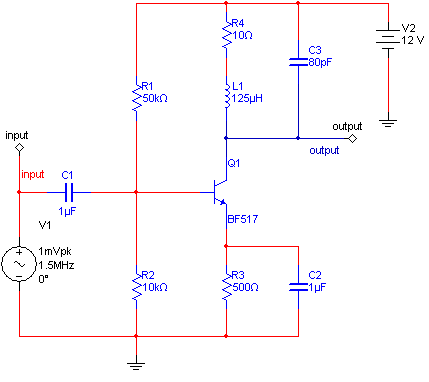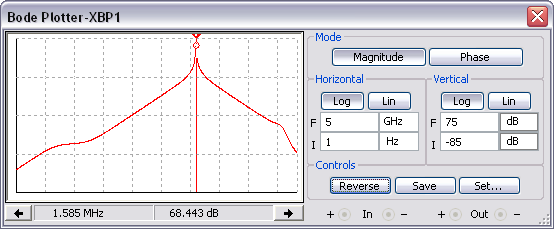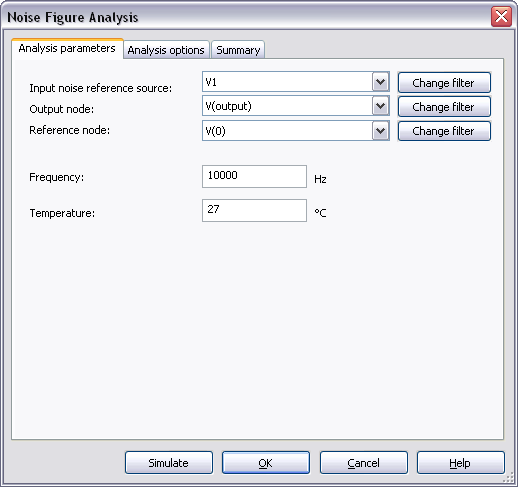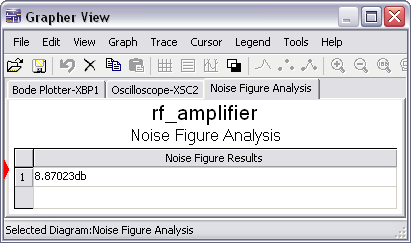# Configuring a Noise Figure Analysis in Multisim

Publish Date: Mar 27, 2012 | 0 Ratings | 0.00 out of 5 | Print | Submit your review

## Overview

Multisim features a comprehensive suite of SPICE analyses for examining circuit behavior. These analyses range from the basic to sophisticated. Each analysis helps you to obtain valuable information such as the effects of component tolerances and sensitivities. For each analysis you need to set settings that will inform Multisim exactly what to analyze, and how.

Multisim simplifies the procedure for an advanced analysis by providing a configuration window. This abstracts away the complexities associated with SPICE syntax and configuration of an analysis. With this window you merely need to specify the parameter values and output nodes of interest.

This tutorial is part of the National Instruments SPICE Analysis Fundamentals Series. Each tutorial in this series provides you with step-by-step instructions on how to configure and run the different SPICE analyses available in Multisim.powerful simulation and analysis while abstracting the complexity of SPICE syntax.

### 1. Introduction

Noise figure is used to specify exactly how noisy a device is. For a transistor, noise figure is simply a measure of how much noise the transistor adds to the signal during the amplification process. In a circuit network, the noise figure is used as a figure of merit to compare the noise in a network with the noise in an ideal or noiseless network. It is a measure of the degradation in signal-to-noise ratio (SNR) between the input and output ports of a network.

Noise figure for a particular component (for instance, a transistor) is provided on the part datasheet. In a SPICE model, the noise figure is specified by the parameter NF. Below is an extract of a SPICE model for a transistor:

.MODEL BF517 NPN (IS=0.480F NF=1.008 BF=99.655 VAF=90.000 IKF=0.190

+ ISE=7.490F NE=1.762 NR=1.010 BR=38.400 VAR=7.000 IKR=93.200M

+ ISC=0.200F NC=1.042

Although noise figure in a model is achieved using a combination of several model parameters, the parameter NF is the most significant value (when simulating noise figure in a transistor model). When selecting a SPICE model to use for Noise Figure Analysis, you must ensure that this parameter exists. If not, the part will operate without noise parameters and therefore will generate no noise.

Multisim calculates the noise figure using the equation:

F = NO / GNS

where:

Multisim prints the noise figure in dB, that is: 10 log10 (F).

### 2. Running Noise Figure Analysis

Consider the RF Amplifier circuit shown in Figure 1. The amplifier is configured for maximum output power at 1.5MHz. You will use Noise Figure Analysis to calculate the noise figure at 10 kHz.Figure 1. RF amplifier circuit.

Complete the following steps to configure and run Noise Figure Analysis:

2. Run the simulation.
3. Use the Bode Plotter to verify the frequency response of the circuit. Using the cursor you can verify that the maximum gain is at approximately at 1.5 MHz (Figure 2).Figure 2. Frequency response.

1. Close the Bode Plotter.
2. Stop the simulation.
3. Select Simulate»Analyses»Noise Figure Analysis. The Noise Figure Analysis window opens. Table 1 describes the Analysis Parameters tab in detail.

Table 1. Parameters used in Noise Figure Analysis.

 Parameter Meaning Input noise reference source Specifies the name of the independent voltage or current source that is to be the input reference source to which equivalent input noise is referred. Output node Specifies the node at which all noise contributions will be summed. Reference node Specifies the reference node for the output noise voltage. Change Filter Displays nodes contained within subcircuits or hierarchical blocks. There are three options: Display internal nodes. Displays nodes within hierarchical blocks and subcircuits. Display submodules. Displays components within semiconductor devices determined by the SPICE model of the semiconductor device. Display open pins. Displays all unconnected nodes of the circuit. Frequency Specifies the frequency for the analysis. Temperature Specifies the temperature for the analysis (typically 27°C).
1. Configure the Analysis Parameters tab as shown in the following figure:Figure 3. Analysis parameters.

1. Click Simulate. The Grapher View opens and displays the result (Figure 4).Figure 4. Noise Figure Analysis result.

Refer to the Noise Analysis tutorial to learn how to calculate the noise spectral density curves.

Entering Expressions in Analyses in Multisim

SPICE Analysis Fundamentals

Circuit Design Technical Library

Join the NI Circuit Design Community